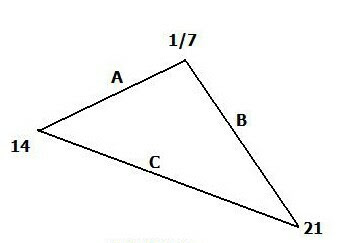## Wednesday, March 18, 20201) For the triangle in Fig. 2, use 7-adic absolute values applied to the sides of the triangle, thereby compute: A, B and C and show it is isosceles
.
Solution:

[A ] 7   =  [14 - 1/7] 7   =  abs {[2 x 7 7   -  1/[1 x 7}

=   abs [1/7 - 7]  =   6 6/7   =  48/7

[B ] 7   =  [1/7 -  21] 7   =   abs {1/ [1 x 7 7   -  [3 x 7}

=   abs [1/7 - 7]  =   6 6/7   =  48/7

[C ] 7   =  [21  -   14] 7   =  abs {[3 x 7 7   -  [2 x 7}

= abs[1/7 - 1/7]  7 =    [0 ] 7   = 1

From these calculations of  (7-adic) absolute values we find side A = side B = 48/7.  Hence in the p-adic format the triangle is isosceles.

2) Find the value of the sum S for: S = 1 + 7 +  (7) 2    + (7) 3   + (7) 4  + (7) 5  + .......

Rewrite multiplying both sides by 7:

7 S =  7 +  (7) 2    + (7) 3   + (7) 4  + (7) 5  + (7) 6  + .......

Subtract the last form from the previous one:

S = 1 + 7 +  (7) 2    + (7) 3   + (7) 4  + (7) 5  + .....

7 S =  7 +  (7) 2    + (7) 3   + (7) 4  +    (7) 5  +  ....
________________________________________

S - 7S = 1 (All other top and bottom terms cancel)

Whence:

-6S = 1 and therefore, S = -1/6

3)  Using (2) as written, "invent" a new irrational number based on the p-adic form.

The most direct way to invent a new p-adic is to employ the same 7-adic form and simply increase powers in a slightly different way..

Then let the new irrational be S'  where S' is a variation of S such that:

S'  =   (7) 3   + (7) 6  + (7) 9  +   + (7) 12  + (7) 15  +.....

Hence, the new irrational S'  is obtained merely by changing the sequence of the powers of 7.